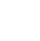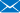Notice of Corporate Name Change -

Adamant Namiki Precision Jewel Co., Ltd., changed its name to Orbray Co., Ltd., effective January 1, 2023.

A special website is available.# Glossary

#### Laser

It stands for Light Amplification of Stimulated Emission of Radiation.

#### Pulse width, pulse duration

Laser emission time of pulse laser.

#### Unit (μ, n, p, f)

μ is 10-6=1/106=1/1000000, n (nano) is 10-9, p (pico) is 10-12, f (femto) is 10-15.

#### Photon energy

Photon energy E[J] is expressed as
E=hc/λ
Where h is the Planck constant 6.626x10-34 [Js], c is the speed of light 3.0x108 [m/s] and λ is the photon's wavelength [m]. As the photon energy is inversely proportional to the wavelength, ultra violet light has higher energy compared to infrared or visible light.

#### Aspect ratio

It expresses the hole shape that calculates by the hole length divided by the hole diameter. It means that the hole length is aspect ratio times larger than the hole diameter. Namely, the hole with large aspect ratio is the long hole against its diameter.

## Contact